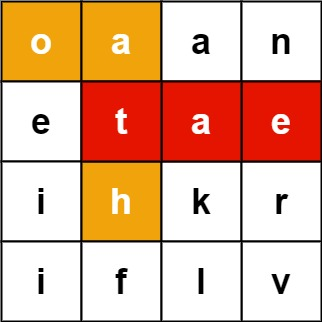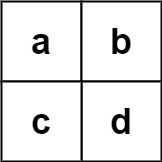#DAY 27
0
AI & Data

## Day 27 LeetCode 212. Word Search II

Given an m x n board of characters and a list of strings words, return all words on the board.

Each word must be constructed from letters of sequentially adjacent cells, where adjacent cells are horizontally or vertically neighboring. The same letter cell may not be used more than once in a word.

### Example 1:``````Input: board = [["o","a","a","n"],["e","t","a","e"],["i","h","k","r"],["i","f","l","v"]], words = ["oath","pea","eat","rain"]
Output: ["eat","oath"]
``````

### Example 2:``````Input: board = [["a","b"],["c","d"]], words = ["abcb"]
Output: []

``````

### Constraints:

``````m == board.length
n == board[i].length
1 <= m, n <= 12
board[i][j] is a lowercase English letter.
1 <= words.length <= 3 * 104
1 <= words[i].length <= 10
words[i] consists of lowercase English letters.
All the strings of words are unique.
``````
``````from typing import List

class Solution:

def dfs(self, board: List[List[str]], word: str, wordid: int, x:int, y:int ):
if x < 0 or y < 0 or x >= len(board) or y >= len(board):
return False

if not board[x][y]:
return False

if  wordid >= len(word):
return False

if board[x][y] != word[wordid]:
return False

if wordid == len(word) -1:
return True

temp = str(board[x][y])
board[x][y] = " "
if self.dfs(board, word, wordid+1, x+1, y) or self.dfs(board, word, wordid+1, x-1, y):
board[x][y] = temp
return True

if self.dfs(board, word, wordid+1, x, y+1) or self.dfs(board, word, wordid+1, x, y-1):
board[x][y] = temp
return True
board[x][y] = temp
return False

def checkin(self, board, word: str):
myset = set()
wset = set()
for a in board:
myset.update(a)
wset.update([i for i in word])

return len(myset & wset) == len(wset)

def findWord(self, board: List[List[str]], word: str) -> List[str]:
for x in range(len(board)):
for y in range(len(board)):
if self.dfs(board, word, 0, x, y):
return True
return False

def findWords(self, board: List[List[str]], words: List[str]) -> List[str]:
result = []
for word in words:
if not self.checkin(board, word):
continue
if self.findWord(board,word):
result.append(word)
return result
pass

board = [["o","a","a","n"],["e","t","a","e"],["i","h","k","r"],["i","f","l","v"]]
words = ["oath","pea","eat","rain"]

print(Solution().findWords(board, words))

board = [["a","b"],["c","d"]]
words = ["abcb"]

print(Solution().findWords(board, words))
``````

### 2 則留言

0

johnting 像是什麼刁鑽的測資?

johnting iT邦新手 5 級 ‧ 2021-10-12 23:59:57 檢舉

0

DFS+IF先判斷 不是就是Backtracking嗎???不會取巧啊

johnting iT邦新手 5 級 ‧ 2021-10-15 22:22:39 檢舉